## Constant Function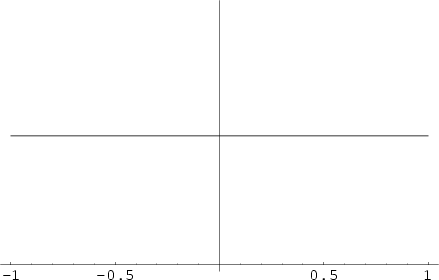A Function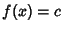which does not change as its parameters vary. The Graph of a 1-D constant Function is a straight Line. The Derivative of a constant Function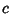is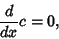(1)

and the Integral is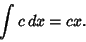(2)

The Fourier Transform of the constant function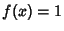is given by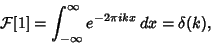(3)

where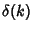is the Delta Function.

Spanier, J. and Oldham, K. B. The Constant Function.'' Ch. 1 in An Atlas of Functions. Washington, DC: Hemisphere, pp. 11-14, 1987.